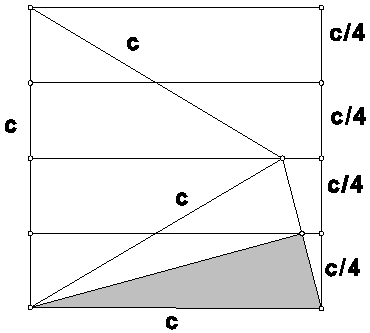# Math 240 Proof without Words #4

M. Flashman

The figure below contains information that can be used to explain why the following statement about the area of a right triangle is true:

If one angle of a right triangle measures 15 degrees, then the area of the triangle is one eighth of the square of the hypotenuse.

Give a written explanation based on the figure by Klara Pinter for the truth of the statement. (Mathematics Magazine, V. 71, No.4, Oct., 1998)

BONUS: Prove the converse: If the area of a right triangle is one eighth of the square of the hypotenuse, then one angle of the triangle measures 15 degrees.